Next: Surface Tension Up: Hydrostatics Previous: Roche Ellipsoids

# Exercises

1. A hollow vessel floats in a basin. If, as a consequence of a leak, water flows slowly into the vessel, how will the level of the water in the basin be affected? (Lamb 1928.)

2. A hollow spherical shell made up of material of specific gravity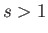has external and internal radii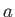and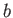, respectively. Demonstrate that the sphere will only float in water if3. Show that the equilibrium of a solid of uniform density floating with an edge or corner just emerging from the water is unstable. (Lamb 1928.)

4. Prove that if a solid of uniform density floats with a flat face just above the waterline then the equilibrium is stable. (Lamb 1928.)

5. Demonstrate that a uniform solid cylinder floating with its axis horizontal is in a stable equilibrium provided that its length exceeds the breadth of the waterline section. [Hint: The cylinder is obviously neutrally stable to rotations about its axis, which means that the corresponding metacentric height is zero.] (Lamb 1928.)

6. Show that a uniform solid cylinder of radiusand heightcan float in stable equilibrium, with its axis vertical, if. If the ratio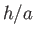exceeds this value, prove that the equilibrium is only stable when the specific gravity of the cylinder lies outside the range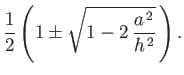7. A uniform, thin, hollow cylinder of radiusand heightis open at both ends. Assuming that, prove that the cylinder cannot float upright if its specific gravity lies in the range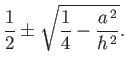(Lamb 1928.)

8. Show that the cylinder of the preceding exercise can float with its axis horizontal providedwhereis the specific gravity of the cylinder. (Lamb 1928.)

9. Prove that any segment of a uniform sphere, made up of a substance lighter than water, can float in stable equilibrium with its plane surface horizontal and immersed. (Lamb 1928.)

10. A vessel carries a tank of oil, of specific gravity, running along its length. Assuming that the surface of the oil is at sea level, show that the effect of the oil's fluidity on the rolling of the vessel is equivalent to a reduction in the metacentric height by, whereis the displacement of the ship,the surface-area of the tank, and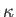the radius of gyration of this area. In what ratio is the effect diminished when a longitudinal partition bisects the tank? (Lamb 1928.)

11. Find the stable equilibrium configurations of a cylinder of elliptic cross-section, with major and minor radiiand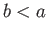, respectively, made up of material of specific gravity, which floats with its axis horizontal.

12. A cylindrical tank has a circular cross-section of radius. Let the center of gravity of the tank be located a distance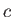above its base. Suppose that the tank is pivoted about a horizontal axis passing through its center of gravity, and is then filled with fluid up to a depthabove its base. Demonstrate that the position in which the tank's axis is upright is unstable for all filling depths provided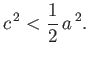Show that if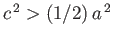then the upright position is stable whenlies in the range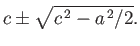13. A thin cylindrical vessel of cross-sectional areafloats upright, being immersed to a depth, and contains water to a depth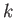. Show that the work required to pump out the water is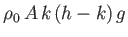. (Lamb 1928.)

14. A sphere of radiusis just immersed in water that is contained in a cylindrical vessel of radius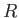whose axis is vertical. Prove that if the sphere is raised just clear of the water then the water's loss of potential energy is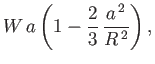whereis the weight of the water originally displaced by the sphere. (Lamb 1928.)

15. A sphere of radius, weight, and specific gravity, rests on the bottom of a cylindrical vessel of radiuswhose axis is vertical, and which contains water to a depth. Show that the work required to lift the sphere out of the vessel is less than if the water had been absent by an amount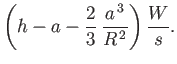(Lamb 1928.)

16. A lead weight is immersed in water that is steadily rotating at an angular velocity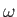about a vertical axis, the weight being suspended from a fixed point on this axis by a string of length. Prove that the position in which the weight hangs vertically downward is stable or unstable depending on whetheror, respectively. Also, show that if the vertical position is unstable then there exists a stable inclined position in which the string is normal to the surface of equal pressure passing though the weight.

17. A thin cylindrical vessel of radiusand height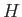is orientated such that its axis is vertical. Suppose that the vessel is filled with liquid of densityto some heightabove the base, spun about its axis at a steady angular velocity, and the liquid allowed to attain a steady state. Demonstrate that, provided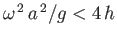and, the net radial thrust on the vertical walls of the vessel is18. A thin cylindrical vessel of radiuswith a plane horizontal lid is just filled with liquid of density, and the whole rotated about a vertical axis at a fixed angular velocity. Prove that the net upward thrust of the fluid on the lid is19. A liquid-filled thin spherical vessel of radiusspins about a vertical diameter at the fixed angular velocity. Assuming that the liquid co-rotates with the vessel, and that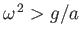, show that the pressure on the wall of the vessel is greatest a depthbelow the center. Also prove that the net normal thrusts on the lower and upper hemispheres areandrespectively, where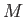is the mass of the liquid.

20. A closed cubic vessel filled with water is rotating about a vertical axis passing through the centers of two opposite sides. Demonstrate that, as a consequence of the rotation, the net thrust on a side is increased bywhereis the length of an edge of the cube, andthe angular velocity of rotation.

21. A closed vessel filled with water is rotating at constant angular velocityabout a horizontal axis. Show that, in the state of relative equilibrium, the constant pressure surfaces in the water are circular cylinders whose common axis is a heightabove the axis of rotation. (Batchelor 2000.)

22. Verify Equations (2.95)-(2.97) and (2.99)-(2.101).

23. Consider a homogeneous, rotating, liquid body of mass, mean radius, and angular velocity, whose outer boundary is a Maclaurin spheroid of eccentricity.
1. Demonstrate thatin the low rotation limit,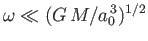. Hence, show that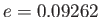for the case of a homogeneous body with the same mass and volume as the Earth, which rotates once every 24 hours.

2. Show that the critical angular velocity at which the bifurcation to the sequence of Jacobi ellipsoids takes place isand occurs when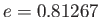. Hence, show that, for the case of a homogeneous body with the same mass and volume as the Earth, the bifurcation would take place at a critical rotation period of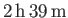.

3. Demonstrate that the maximum angular velocity consistent with a spheroidal shape is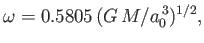and occurs when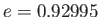. Hence, show that, for the case of a homogeneous body with the same mass and volume as the Earth, this maximum velocity corresponds to a minimum rotation period of.Next: Surface Tension Up: Hydrostatics Previous: Roche Ellipsoids
Richard Fitzpatrick 2016-03-31